Intermediate Algebra 2e

# 3.4Graph Linear Inequalities in Two Variables

Intermediate Algebra 2e3.4 Graph Linear Inequalities in Two Variables

### Learning Objectives

By the end of this section, you will be able to:

• Verify solutions to an inequality in two variables.
• Recognize the relation between the solutions of an inequality and its graph.
• Graph linear inequalities in two variables
• Solve applications using linear inequalities in two variables

### Be Prepared 3.10

Before you get started, take this readiness quiz.

Graph $x>2x>2$ on a number line.
If you missed this problem, review Example 2.48.

### Be Prepared 3.11

Solve: $4x+3>234x+3>23$.
If you missed this problem, review Example 2.52.

### Be Prepared 3.12

Translate: $8>x>38>x>3$.
If you missed this problem, review Example 2.56.

### Verify Solutions to an Inequality in Two Variables

Previously we learned to solve inequalities with only one variable. We will now learn about inequalities containing two variables. In particular we will look at linear inequalities in two variables which are very similar to linear equations in two variables.

### Linear Inequality

A linear inequality is an inequality that can be written in one of the following forms:

$Ax+By>CAx+By≥CAx+ByCAx+By≥CAx+By

Where A and B are not both zero.

Recall that an inequality with one variable had many solutions. For example, the solution to the inequality $x>3x>3$ is any number greater than 3. We showed this on the number line by shading in the number line to the right of 3, and putting an open parenthesis at 3. See Figure 3.10.

Figure 3.10

Similarly, linear inequalities in two variables have many solutions. Any ordered pair $(x,y)(x,y)$ that makes an inequality true when we substitute in the values is a solution to a linear inequality.

### Solution to a Linear Inequality

An ordered pair $(x,y)(x,y)$ is a solution to a linear inequality if the inequality is true when we substitute the values of x and y.

### Example 3.34

Determine whether each ordered pair is a solution to the inequality $y>x+4:y>x+4:$

$(0,0)(0,0)$ $(1,6)(1,6)$ $(2,6)(2,6)$ $(−5,−15)(−5,−15)$ $(−8,12)(−8,12)$

### Try It 3.67

Determine whether each ordered pair is a solution to the inequality $y>x−3:y>x−3:$

$(0,0)(0,0)$ $(4,9)(4,9)$ $(−2,1)(−2,1)$ $(−5,−3)(−5,−3)$ $(5,1)(5,1)$

### Try It 3.68

Determine whether each ordered pair is a solution to the inequality $y

$(0,0)(0,0)$ $(8,6)(8,6)$ $(−2,−1)(−2,−1)$ $(3,4)(3,4)$ $(−1,−4)(−1,−4)$

### Recognize the Relation Between the Solutions of an Inequality and its Graph

Now, we will look at how the solutions of an inequality relate to its graph.

Let’s think about the number line in shown previously again. The point $x=3x=3$ separated that number line into two parts. On one side of 3 are all the numbers less than 3. On the other side of 3 all the numbers are greater than 3. See Figure 3.11.

Figure 3.11 The solution to $x>3x>3$ is the shaded part of the number line to the right of $x=3.x=3.$

Similarly, the line $y=x+4y=x+4$ separates the plane into two regions. On one side of the line are points with $y On the other side of the line are the points with $y>x+4.y>x+4.$ We call the line $y=x+4y=x+4$ a boundary line.

### Boundary Line

The line with equation $Ax+By=CAx+By=C$ is the boundary line that separates the region where $Ax+By>CAx+By>C$ from the region where $Ax+By

For an inequality in one variable, the endpoint is shown with a parenthesis or a bracket depending on whether or not a is included in the solution: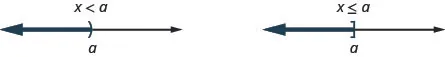Similarly, for an inequality in two variables, the boundary line is shown with a solid or dashed line to show whether or not it the line is included in the solution.

$Ax+ByCAx+By≥C Boundary line isAx+By=CBoundary line isAx+By=C Boundary line is not included in solution.Boundary line is included in solution. Boundary line is dashed.Boundary line is solid.Ax+ByCAx+By≥C Boundary line isAx+By=CBoundary line isAx+By=C Boundary line is not included in solution.Boundary line is included in solution. Boundary line is dashed.Boundary line is solid.$

Now, let’s take a look at what we found in Example 3.34. We’ll start by graphing the line $y=x+4,y=x+4,$ and then we’ll plot the five points we tested, as shown in the graph. See Figure 3.12.

Figure 3.12

In Example 3.34 we found that some of the points were solutions to the inequality $y>x+4y>x+4$ and some were not.

Which of the points we plotted are solutions to the inequality $y>x+4?y>x+4?$

The points $(1,6)(1,6)$ and $(−8,12)(−8,12)$ are solutions to the inequality $y>x+4.y>x+4.$ Notice that they are both on the same side of the boundary line $y=x+4.y=x+4.$

The two points $(0,0)(0,0)$ and $(−5,−15)(−5,−15)$ are on the other side of the boundary line $y=x+4,y=x+4,$ and they are not solutions to the inequality $y>x+4.y>x+4.$ For those two points, $y

What about the point $(2,6)?(2,6)?$ Because $6=2+4,6=2+4,$ the point is a solution to the equation $y=x+4,y=x+4,$ but not a solution to the inequality $y>x+4.y>x+4.$ So the point $(2,6)(2,6)$ is on the boundary line.

Let’s take another point above the boundary line and test whether or not it is a solution to the inequality $y>x+4.y>x+4.$ The point $(0,10)(0,10)$ clearly looks to above the boundary line, doesn’t it? Is it a solution to the inequality?

$y>x+410>?0+410>4y>x+410>?0+410>4$

So, $(0,10)(0,10)$ is a solution to $y>x+4.y>x+4.$

Any point you choose above the boundary line is a solution to the inequality $y>x+4.y>x+4.$ All points above the boundary line are solutions.

Similarly, all points below the boundary line, the side with $(0,0)(0,0)$ and $(−5,−15),(−5,−15),$ are not solutions to $y>x+4,y>x+4,$ as shown in Figure 3.13.

Figure 3.13

The graph of the inequality $y>x+4y>x+4$ is shown in below.

The line $y=x+4y=x+4$ divides the plane into two regions. The shaded side shows the solutions to the inequality $y>x+4.y>x+4.$

The points on the boundary line, those where $y=x+4,y=x+4,$ are not solutions to the inequality $y>x+4,y>x+4,$ so the line itself is not part of the solution. We show that by making the line dashed, not solid.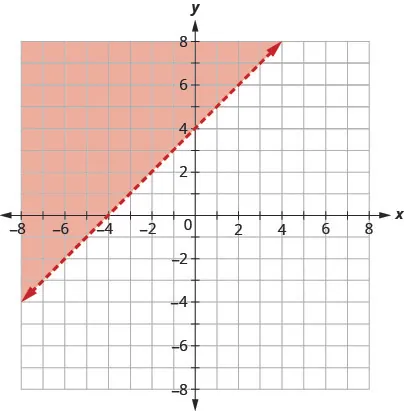### Example 3.35

The boundary line shown in this graph is $y=2x−1.y=2x−1.$ Write the inequality shown by the graph.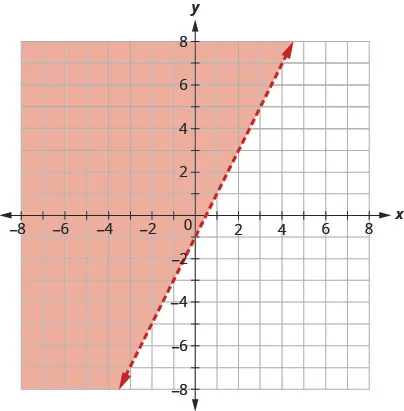### Try It 3.69

Write the inequality shown by the graph with the boundary line $y=−2x+3.y=−2x+3.$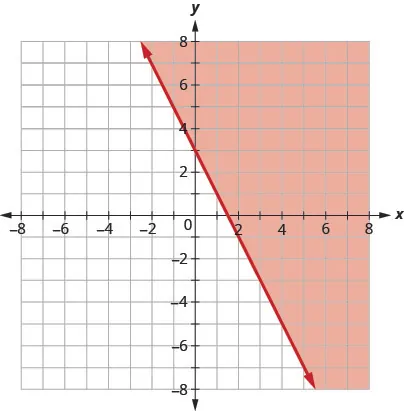### Try It 3.70

Write the inequality shown by the graph with the boundary line $y=12x−4.y=12x−4.$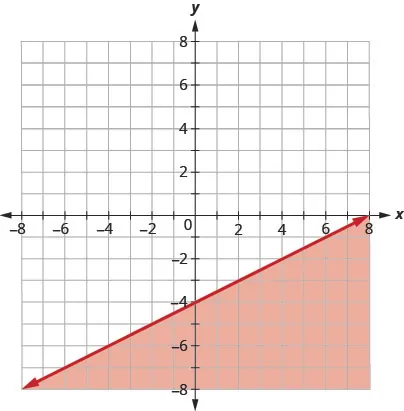### Example 3.36

The boundary line shown in this graph is $2x+3y=6.2x+3y=6.$ Write the inequality shown by the graph.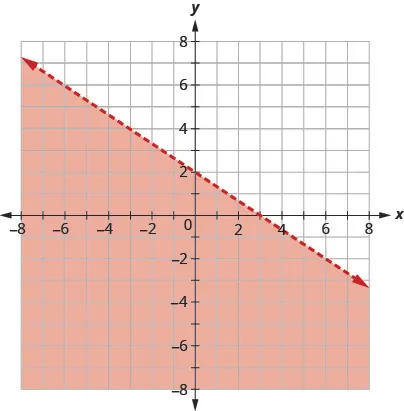### Try It 3.71

Write the inequality shown by the shaded region in the graph with the boundary line $x−4y=8.x−4y=8.$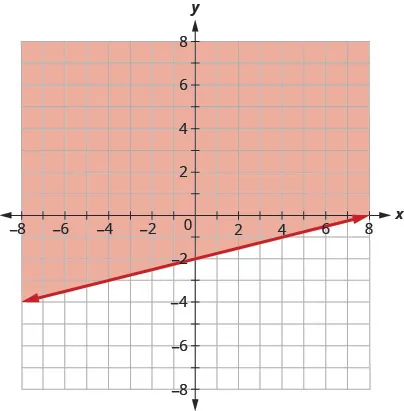### Try It 3.72

Write the inequality shown by the shaded region in the graph with the boundary line $3x−y=6.3x−y=6.$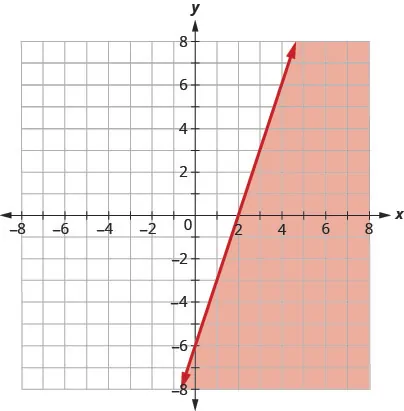### Graph Linear Inequalities in Two Variables

Now that we know what the graph of a linear inequality looks like and how it relates to a boundary equation we can use this knowledge to graph a given linear inequality.

### Example 3.37

#### How to Graph a Linear Equation in Two Variables

Graph the linear inequality $y≥34x−2.y≥34x−2.$

### Try It 3.73

Graph the linear inequality $y≥52x−4.y≥52x−4.$

### Try It 3.74

Graph the linear inequality $y<23x−5.y<23x−5.$

The steps we take to graph a linear inequality are summarized here.

### How To

#### Graph a linear inequality in two variables.

1. Step 1.
Identify and graph the boundary line.
• If the inequality is $≤or≥,≤or≥,$ the boundary line is solid.
• If the inequality is $,,$ the boundary line is dashed.
2. Step 2. Test a point that is not on the boundary line. Is it a solution of the inequality?
3. Step 3.
Shade in one side of the boundary line.
• If the test point is a solution, shade in the side that includes the point.
• If the test point is not a solution, shade in the opposite side.

### Example 3.38

Graph the linear inequality $x−2y<5.x−2y<5.$

### Try It 3.75

Graph the linear inequality: $2x−3y<6.2x−3y<6.$

### Try It 3.76

Graph the linear inequality: $2x−y>3.2x−y>3.$

What if the boundary line goes through the origin? Then, we won’t be able to use $(0,0)(0,0)$ as a test point. No problem—we’ll just choose some other point that is not on the boundary line.

### Example 3.39

Graph the linear inequality: $y≤​−4x.y≤​−4x.$

### Try It 3.77

Graph the linear inequality: $y>−3x.y>−3x.$

### Try It 3.78

Graph the linear inequality: $y≥−2x.y≥−2x.$

Some linear inequalities have only one variable. They may have an x but no y, or a y but no x. In these cases, the boundary line will be either a vertical or a horizontal line.

Recall that:

$x=avertical line y=bhorizontal line x=avertical line y=bhorizontal line$

### Example 3.40

Graph the linear inequality: $y>3.y>3.$

### Try It 3.79

Graph the linear inequality: $y<5.y<5.$

### Try It 3.80

Graph the linear inequality: $y≤−1.y≤−1.$

### Solve Applications using Linear Inequalities in Two Variables

Many fields use linear inequalities to model a problem. While our examples may be about simple situations, they give us an opportunity to build our skills and to get a feel for how they might be used.

### Example 3.41

Hilaria works two part time jobs in order to earn enough money to meet her obligations of at least $240 a week. Her job in food service pays$10 an hour and her tutoring job on campus pays $15 an hour. How many hours does Hilaria need to work at each job to earn at least$240?

Let $xx$ be the number of hours she works at the job in food service and let y be the number of hours she works tutoring. Write an inequality that would model this situation.

Graph the inequality.

Find three ordered pairs $(x,y)(x,y)$ that would be solutions to the inequality. Then, explain what that means for Hilaria.

### Try It 3.81

Hugh works two part time jobs. One at a grocery store that pays $10 an hour and the other is babysitting for$13 hour. Between the two jobs, Hugh wants to earn at least $260 a week. How many hours does Hugh need to work at each job to earn at least$260?

Let x be the number of hours he works at the grocery store and let y be the number of hours he works babysitting. Write an inequality that would model this situation.

Graph the inequality.

Find three ordered pairs (x, y) that would be solutions to the inequality. Then, explain what that means for Hugh.

### Try It 3.82

Veronica works two part time jobs in order to earn enough money to meet her obligations of at least $280 a week. Her job at the day spa pays$10 an hour and her administrative assistant job on campus pays $17.50 an hour. How many hours does Veronica need to work at each job to earn at least$280?

Let x be the number of hours she works at the day spa and let y be the number of hours she works as administrative assistant. Write an inequality that would model this situation.

Graph the inequality.

Find three ordered pairs (x, y) that would be solutions to the inequality. Then, explain what that means for Veronica

### Media

Access this online resource for additional instruction and practice with graphing linear inequalities in two variables.

### Section 3.4 Exercises

#### Practice Makes Perfect

Verify Solutions to an Inequality in Two Variables

In the following exercises, determine whether each ordered pair is a solution to the given inequality.

237.

Determine whether each ordered pair is a solution to the inequality $y>x−1:y>x−1:$

$(0,1)(0,1)$
$(−4,−1)(−4,−1)$
$(4,2)(4,2)$
$(3,0)(3,0)$
$(−2,−3)(−2,−3)$

238.

Determine whether each ordered pair is a solution to the inequality $y>x−3:y>x−3:$

$(0,0)(0,0)$
$(2,1)(2,1)$
$(−1,−5)(−1,−5)$
$(−6,−3)(−6,−3)$
$(1,0)(1,0)$

239.

Determine whether each ordered pair is a solution to the inequality $y<3x+2:y<3x+2:$

$(0,3)(0,3)$
$(−3,−2)(−3,−2)$
$(−2,0)(−2,0)$
$(0,0)(0,0)$
$(−1,4)(−1,4)$

240.

Determine whether each ordered pair is a solution to the inequality $y<−2x+5:y<−2x+5:$

$(−3,0)(−3,0)$
$(1,6)(1,6)$
$(−6,−2)(−6,−2)$
$(0,1)(0,1)$
$(5,−4)(5,−4)$

241.

Determine whether each ordered pair is a solution to the inequality $3x−4y>4:3x−4y>4:$

$(5,1)(5,1)$
$(−2,6)(−2,6)$
$(3,2)(3,2)$
$(10,−5)(10,−5)$
$(0,0)(0,0)$

242.

Determine whether each ordered pair is a solution to the inequality $2x+3y>2:2x+3y>2:$

$(1,1)(1,1)$
$(4,−3)(4,−3)$
$(0,0)(0,0)$
$(−8,12)(−8,12)$
$(3,0)(3,0)$

Recognize the Relation Between the Solutions of an Inequality and its Graph

In the following exercises, write the inequality shown by the shaded region.

243.

Write the inequality shown by the graph with the boundary line $y=3x−4.y=3x−4.$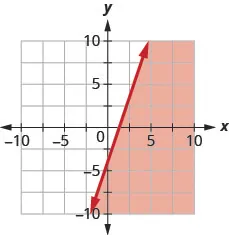244.

Write the inequality shown by the graph with the boundary line $y=2x−4.y=2x−4.$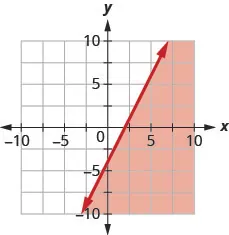245.

Write the inequality shown by the graph with the boundary line $y=12x+1.y=12x+1.$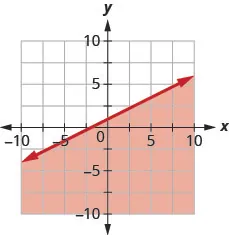246.

Write the inequality shown by the graph with the boundary line $y=−13x−2.y=−13x−2.$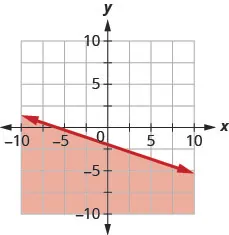247.

Write the inequality shown by the shaded region in the graph with the boundary line $x+y=5.x+y=5.$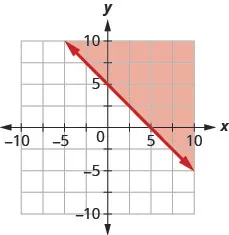248.

Write the inequality shown by the shaded region in the graph with the boundary line $x+y=3.x+y=3.$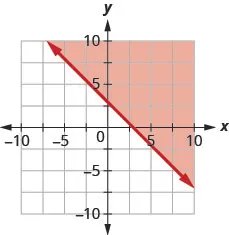249.

Write the inequality shown by the shaded region in the graph with the boundary line $3x−y=6.3x−y=6.$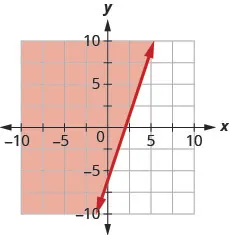250.

Write the inequality shown by the shaded region in the graph with the boundary line $2x−y=4.2x−y=4.$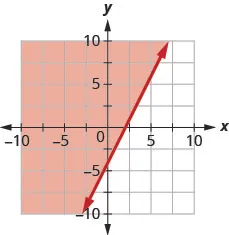Graph Linear Inequalities in Two Variables

In the following exercises, graph each linear inequality.

251.

Graph the linear inequality: $y>23x−1.y>23x−1.$

252.

Graph the linear inequality: $y<35x+2.y<35x+2.$

253.

Graph the linear inequality: $y≤−12x+4.y≤−12x+4.$

254.

Graph the linear inequality: $y≥−13x−2.y≥−13x−2.$

255.

Graph the linear inequality: $x−y≤3.x−y≤3.$

256.

Graph the linear inequality: $x−y≥−2.x−y≥−2.$

257.

Graph the linear inequality: $4x+y>−4.4x+y>−4.$

258.

Graph the linear inequality: $x+5y<−5.x+5y<−5.$

259.

Graph the linear inequality: $3x+2y≥−6.3x+2y≥−6.$

260.

Graph the linear inequality: $4x+2y≥−8.4x+2y≥−8.$

261.

Graph the linear inequality: $y>4x.y>4x.$

262.

Graph the linear inequality: $y≤−3x.y≤−3x.$

263.

Graph the linear inequality: $y<−10.y<−10.$

264.

Graph the linear inequality: $y≥2.y≥2.$

265.

Graph the linear inequality: $x≤5.x≤5.$

266.

Graph the linear inequality: $x≥0.x≥0.$

267.

Graph the linear inequality: $x−y<4.x−y<4.$

268.

Graph the linear inequality: $x−y<−3.x−y<−3.$

269.

Graph the linear inequality: $y≥32x.y≥32x.$

270.

Graph the linear inequality: $y≤54x.y≤54x.$

271.

Graph the linear inequality: $y>−2x+1.y>−2x+1.$

272.

Graph the linear inequality: $y<−3x−4.y<−3x−4.$

273.

Graph the linear inequality: $2x+y≥−4.2x+y≥−4.$

274.

Graph the linear inequality: $x+2y≤−2.x+2y≤−2.$

275.

Graph the linear inequality: $2x−5y>10.2x−5y>10.$

276.

Graph the linear inequality: $4x−3y>12.4x−3y>12.$

Solve Applications using Linear Inequalities in Two Variables

277.

Harrison works two part time jobs. One at a gas station that pays $11 an hour and the other is IT troubleshooting for $16.5016.50$ an hour. Between the two jobs, Harrison wants to earn at least$330 a week. How many hours does Harrison need to work at each job to earn at least $330? Let x be the number of hours he works at the gas station and let y be the number of (hours he works troubleshooting. Write an inequality that would model this situation. Graph the inequality. Find three ordered pairs $(x,y)(x,y)$ that would be solutions to the inequality. Then, explain what that means for Harrison. 278. Elena needs to earn at least$450 a week during her summer break to pay for college. She works two jobs. One as a swimming instructor that pays $9 an hour and the other as an intern in a genetics lab for$22.50 per hour. How many hours does Elena need to work at each job to earn at least \$450 per week?

Let x be the number of hours she works teaching swimming and let y be the number of hours she works as an intern. Write an inequality that would model this situation.

Graph the inequality.

Find three ordered pairs $(x,y)(x,y)$ that would be solutions to the inequality. Then, explain what that means for Elena.

279.

The doctor tells Laura she needs to exercise enough to burn 500 calories each day. She prefers to either run or bike and burns 15 calories per minute while running and 10 calories a minute while biking.

If x is the number of minutes that Laura runs and y is the number minutes she bikes, find the inequality that models the situation.

Graph the inequality.

List three solutions to the inequality. What options do the solutions provide Laura?

280.

Armando’s workouts consist of kickboxing and swimming. While kickboxing, he burns 10 calories per minute and he burns 7 calories a minute while swimming. He wants to burn 600 calories each day.

If x is the number of minutes that Armando will kickbox and y is the number minutes he will swim, find the inequality that will help Armando create a workout for today.

Graph the inequality.

List three solutions to the inequality. What options do the solutions provide Armando?

#### Writing Exercises

281.

Lester thinks that the solution of any inequality with a $>>$ sign is the region above the line and the solution of any inequality with a $<<$ sign is the region below the line. Is Lester correct? Explain why or why not.

282.

Explain why, in some graphs of linear inequalities, the boundary line is solid but in other graphs it is dashed.

#### Self Check

After completing the exercises, use this checklist to evaluate your mastery of the objectives of this section.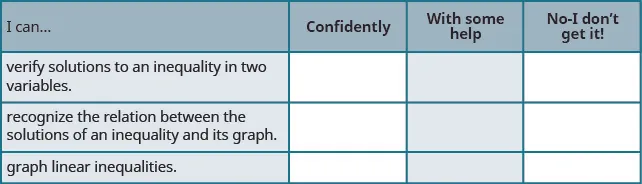On a scale of 1–10, how would you rate your mastery of this section in light of your responses on the checklist? How can you improve this?

Order a print copy

As an Amazon Associate we earn from qualifying purchases.